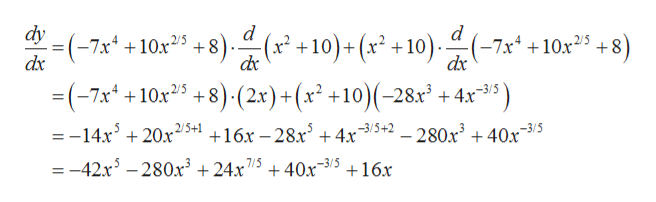# Differentiate the following function:  y= (-7x^4 + 10x^2/5 + 8) (x^2 + 10)

Question
1 views

Differentiate the following function:

y= (-7x^4 + 10x^2/5 + 8) (x^2 + 10)

check_circle

Step 1

The given expression is:

Step 2

Formula used:

Step 3

Differentiating the function y bot...help_outlineImage Transcriptionclosedy = (-7x4 + 10x25 +8 dx d -7x410x25+8 dx 8). dr 10)+(x* +10 (-7x10x2 +8)(2x)+(x +10)(-28x +4x3 2/5 =-14x3 20x25+1 +16x-28x5 + 4x35+2 - 280x3 + 40x .-3/5 = -42x5 -280x3 +24x 7/5 +40x3516x fullscreen

### Want to see the full answer?

See Solution

#### Want to see this answer and more?

Solutions are written by subject experts who are available 24/7. Questions are typically answered within 1 hour.*

See Solution
*Response times may vary by subject and question.
Tagged in

### Derivative